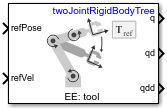Model rigid body tree motion given task-space inputs

• Library:
• Robotics System Toolbox / Manipulator Algorithms

•## Description

The Task Space Motion Model block models the closed-loop task-space motion of a manipulator, specified as a `rigidBodyTree` object. The motion model behavior is defined using proportional-derivative (PD) control.

For more details about the equations of motion, see Task-Space Motion Model.

## Ports

### Input

expand all

Homogenous transformation matrix representing the desired end effector pose, specified in meters.

6-element vector representing the desired linear and angular velocities of the end effector, specified in meters per second and radians per second.

6-by-m matrix representing external forces, specified in meters per second. m is the number of bodies in the `rigidBodyTree` object in the ```Rigid body tree``` parameter.

#### Dependencies

To enable this port, set the ```Show external force input``` parameter to `on`.

### Output

expand all

Joint positions output as an n-element vector in radians or meters, where n is the number of nonfixed joints in the `rigidBodyTree` object in the ```Rigid body tree``` parameter.

Joint velocities output as an n-element vector in radians per second or meters per second, where n is the number of nonfixed joints in the `rigidBodyTree` object in the ```Rigid body tree``` parameter.

Joint accelerations output as an n-element in radians per second squared or meters per second squared, where n is the number of nonfixed joints in the `rigidBodyTree` object in the ```Rigid body tree``` parameter.

## Parameters

expand all

Robot model, specified as a `RigidBodyTree` object. You can also import a robot model from an URDF (Unified Robot Description Formation) file using `importrobot`.

The default robot model, `twoJointRigidBodyTree`, is a robot with revolute joints and two degrees of freedom.

This parameter defines the body that will be used as the end effector, and for which the task space motion is defined. The property must correspond to a body name in the `rigidBodyTree` object of the property. Click to select a body from the `rigidBodyTree`. If the `rigidBodyTree` is updated without also updating the end effector, the body with the highest index is assigned by default.

Proportional gain for proportional-derivative (PD) control, specified as a 6-by-6 matrix.

Derivative gain for proportional-derivative (PD) control, specified as a 6-by-6 matrix.

Damping ratios on each joint, specified as a scalar or n-element vector, where n is the number of nonfixed joints in the `rigidBodyTree` object in the ```Rigid body tree``` parameter.

Click the check-box to enable this parameter to input external forces using the `FExt` port.

Initial joint positions, specified as a n-element vector or scalar in radians. n is the number of nonfixed joints in the `rigidBodyTree` object in the ```Rigid body tree``` parameter.

Initial joint velocities, specified as a n-element vector or scalar in radians per second. n is the number of nonfixed joints in the `rigidBodyTree` object in the ```Rigid body tree``` parameter.

• `Interpreted execution` — Simulate model using the MATLAB® interpreter. For more information, see Simulation Modes (Simulink).

• `Code generation` — Simulate model using generated C code. The first time you run a simulation, Simulink® generates C code for the block. The C code is reused for subsequent simulations, as long as the model does not change.

Tunable: No

 Craig, John J. Introduction to Robotics: Mechanics and Control. Upper Saddle River, NJ: Pearson Education, 2005.

 Spong, Mark W., Seth Hutchinson, and Mathukumalli Vidyasagar. Robot Modeling and Control. Hoboken, NJ: Wiley, 2006.